# Online Civil Engineering Test - Previous GATE Exam Papers Test 1Loading Test...

Instruction:

• This is a FREE online test. DO NOT pay money to anyone to attend this test.
• Total number of questions : 20.
• Time alloted : 30 minutes.
• Each question carry 1 mark, no negative marks.
• DO NOT refresh the page.
• All the best :-).

1.

A two span beam with an internal hinge is shown below.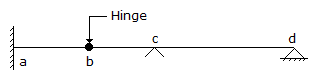The conjugate beam corresponding to this beam is

A.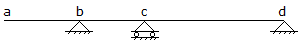B.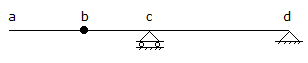C.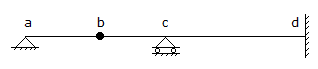D.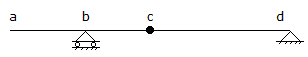2.

In reinforced concrete, pedestal is defined as compression member, whose effective length does not exceed its least dimension by

A.
 12 timesB.
 3 timesC.
 16 timesD.
 8 times3.

For the frame shown in the below figure, the maximum bending moment in the column is,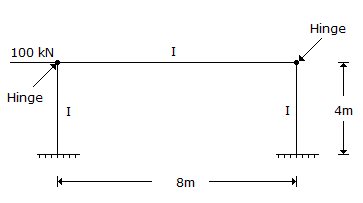A.
 zeroB.
 400 kNmC.
 100 kNmD.
 200 kNm4.

The reaction time for calculation of stopping distance may be assumed as

A.
 5 secsB.
 2.5 secsC.
 0.5 secsD.
 10.0 secs5.

The following characteristics pertain to the sand filters used in water industry.

1. Filtration rate is 1 to 4 m3 (m2 day).
2. Typical duration of operation in one run is 24 to 72 hours.
3. Operating cost is low.

Which of the above characteristics pertain to slow sand filters ?

A.
 I, II and IIIB.
 I and IIC.
 II and IIID.
 I and III6.

If an element of a stressed body is in a state of pure shear with a magnitude of 80 N/mm2 , the magnitude of maximum principal stress at that location is

A.
 80 N/mm2B.
 113.14 N/mm2C.
 120 N/mm2D.
 56.57 N/mm27.

In deriving the equation for the hydraulic jump in a rectangular channel in terms of the conjugate depths and the initial Froude number.

A.
 continuity equation and energy equation are usedB.
 continuity equation and momentum equation are usedC.
 equations of continuity, mementum and energy are usedD.
 gradually varied flow equation is used8.

A septic tank is

A.
 an aerobic method of on site sewage treatmentB.
 an anaerobic method of on site treatmentC.
 a physical method of water treatmentD.
 a physicochemical method of water treatment9.

The members EJ and IJ of steel truss shown in the figure below are subjected to a temperature rise of 30° C. The coefficient of thermal expansion of steel is 0.000012 per °C per unit length. The displacement (mm) of joint E relative to joint H along the direction HE of the truss, is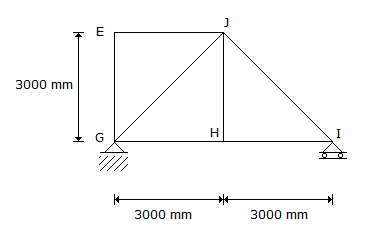A.
 0.255B.
 0.589C.
 0.764D.
 1.02610.

Water flows at a depth of 0.1 m with a velocity of 6 m/s in a rectangular channel. The alternate dpeth is

A.
 0.30 mB.
 0.40 mC.
 0.86 mD.
 0.81 m11.

An isochrone is a line on the basin map

A.
 Joining raingauge stations having equal rainfall-durationB.
 Joining points having equal rainfall depth in a given time intervalC.
 Joining points having equal time of travel of surface runoff to the catchment outletD.
 Joining points which are at equal distance from the catchment outlet12.

Rotational stiffness-coefficient, K11 for the frame having two members of equal EI/l is given by :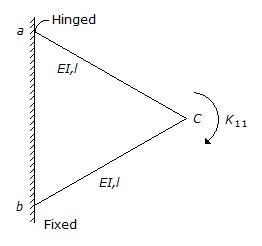A.
 5 EI/lB.
 6 EI/lC.
 7 EI/lD.
 8 EI/l13.

Chemical oxygen Demand (COD) of a sample is always greater than Biochemical Oxygen Demand (BOD) since it represents :

A.
 biodegradable organic matter onlyB.
 biodegradable and non-biodegradable organic matterC.
 non-biodegradable organic matterD.
 inorganic matter14.

The equation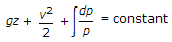along a stream line holds true for :

A.
 steady, frictionless, compressible fluidB.
 steady, uniform, incompressible fluidC.
 steady, frictionless, incompressible fluidD.
 unsteady incompressible fluid15.

According to Darcy's law for flow through porous media, the velocity is proportional to

A.
 Effective stressB.
 hydraulic gradientC.
 cohesionD.
 stability number16.

Factor of safety adopted by IS : 800 - 1984 while arriving at the permissible stress in axial compression, is :

A.
 2B.
 1C.
 1.67D.
 1.517.

Bending moments at joint b and c of the portal frame are respectively :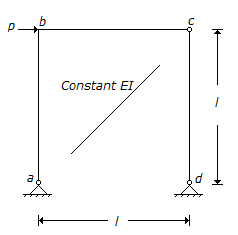A.
 + p l/2, - p l/2B.
 + p l/2, + p l/2C.
 + p l/4, - p l/4D.
 + p l/4, + p l/418.

Water table drops by 3 m in an irrigable land of 50 hectare. If porosity and specific retention are 0.30 and 0.10 respectively, the change in storage in hectare-meter is :

A.
 15B.
 30C.
 45D.
 6019.

Mechanical stabilization requires :

A.
 mixing of two or more types of natural soilsB.
 addition of chemicals to soilsC.
 addition of lime to soilsD.
 addition of cementing material to soils20.

The flow of water (mass density = 1000 kg/m3 and kinematic viscosity = 10-6 m2/s) in a commercial pipe, having equivalent roughness ks as 0.12 mm, yields an average shear stress at the pipe boundary = 600 N/m2. The value of ks/δ' (δ' being the thickness of laminar sub-layer) for this pipe is

A.
 0.25B.
 0.5C.
 6D.
 8